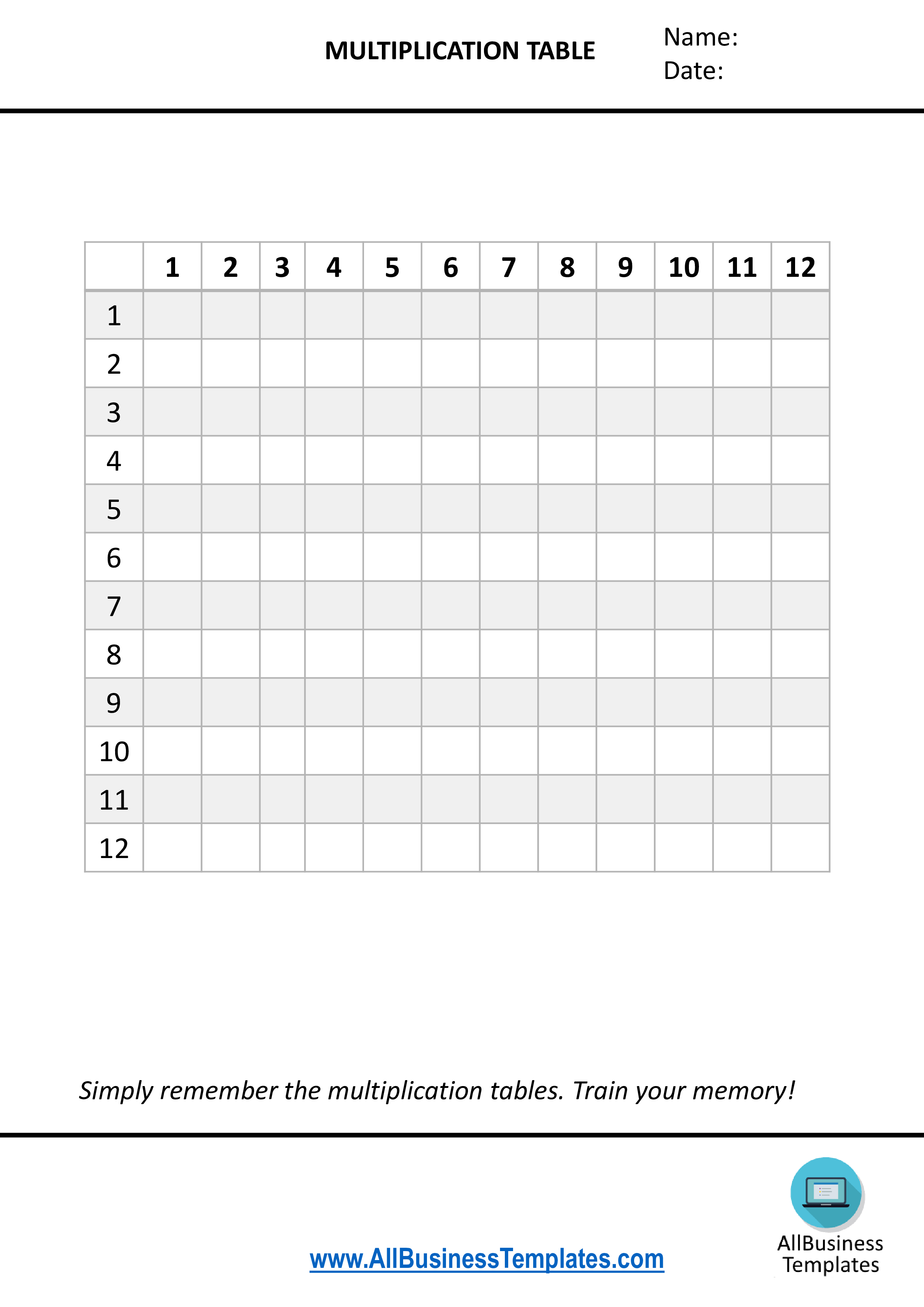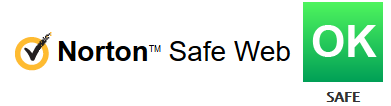# Multiplication TablesHaga clic en la imagen para ampliar / Haga clic en el botón de abajo para ver más imágenes

## Guardar, completar los espacios en blanco, imprimir, listo!What's the best way to learn to multiply? Check out this 'Learn how to multiplication tables educational template now!

Formatos de archivo disponibles:

.pdf

• Este documento ha sido certificado por un profesionall
• 100% personalizable

Calificación de la plantilla： 8

Malware en virus vrij：Education Educación math mates math for kids matemáticas para niños mathematics matemáticas multiply multiplicar Com Printable Multiplication Tables Tablas de multiplicar imprimibles Multiplication Times Tables Tablas de multiplicar tiempos Time Table Chart Tabla de Horarios Mthws Allfreeprintable Todo libre de fuentes multiplication table tabla de multiplicación multiplication table worksheet tabla de tablas de multiplicar education times table tabla de multiplicar la educación printable multiplication times tables tablas de multiplicar para imprimir times tables tablas de multiplicar time table tabla de tiempo math for dummies Matemáticas para los maniquíes math for beginners matemáticas para principiantes learn multiplication aprender la multiplicación learn to calculate aprender a calcular how to multiply como multiplicar multiplication education educación de multiplicación multiplication table for kids printable multiplication table multiplication table games multiplication tables from 1 to 12 multiplication table multiplication tables multiplication table printable multiplication table chart printable multiplication table 12 multiplication table multiplication table up to 100 math mathematics multiplication table up to printable multiplication chart memorize multiplication tables multiplication table 12 multiplication tables to 20 memorize multiplication tables

What's the best way to learn to multiply? Check out this 'Learn how to multiply tables template now!

In math, the multiplication table is a a common mathematical table that is useful to memorize the tables, often from 1 to 12 for children. It defines the multiplication operation for an algebraic system.

Learning math through play is a very good way of entertainment for parents and kids together since it gives your child a nice experience and enables them to learn how to recognize and understand how to multiply.  Through multiplication these days, together with the parent, the child can develop social and cognitive skills, mature emotionally and gain the self-confidence required to engage in new experiences and environments.

Keyways with such learning to multiply by educational templates is that children learn include playing, being with other people, being active, ideas and experiences, communication with others, meeting mental challenges, being shown how to do new things, practicing and repeating skills and having fun.

Using this learning how to multiply template guarantees your family a fun learning experience that stimulates the brain and memory of your child(ren) and yourself!

Nada en este sitio se considerará asesoramiento legal y no se establece una relación abogado-cliente.

Deja una respuesta. Si tiene preguntas o comentarios, puede colocarlos a continuación.